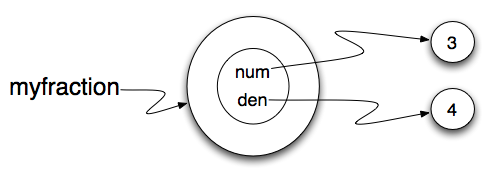To design our class, we simply need to use the analysis above to realize that the state of a fraction object can be completely described by representing two integers. We can begin by implementing the Fraction class and the __init__ method which will allow the user to provide a numerator and a denominator for the fraction being created.
Note that the __str__ method provides a “typical” looking fraction using a slash between the numerator and denominator. The figure below shows the state of myfraction. We have also added a few simple accessor methods, getNum and getDen, that can return the state values for the fraction.